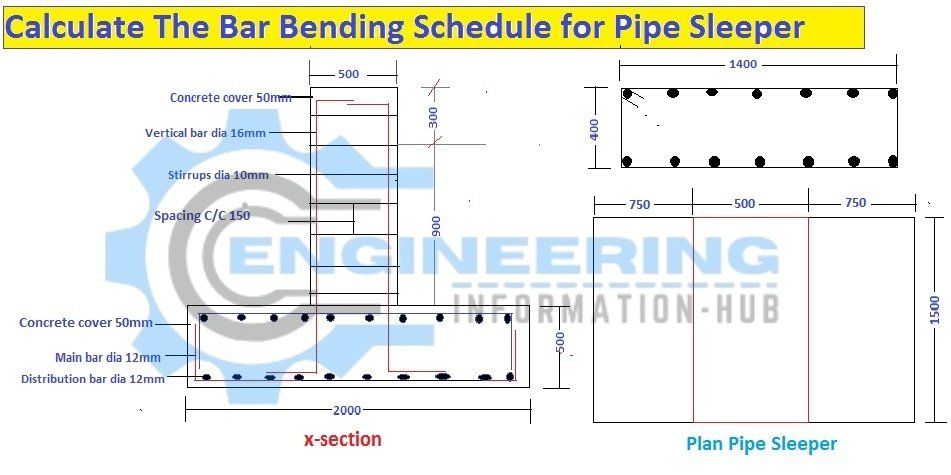Bar Bending Schedule

# Bar Bending Schedule for The Pipe Sleeper

Today I have a topic on how to calculate the bar bending schedule for pipe sleepers. Uses of pipe sleeper and what is the pipe sleeper.Bar Bending Schedule for The Pipe Sleeper.

### What is the Pipe sleeper?

Any pipeline sleeper for just a Supports for Pipe Line. Pipe Sleeper Foundation Use the Pipe Line Supports they have a Base Plate on the top Side that Plate is used for holding the Pipe with the help of any Coupling this coupling is welded with the Base Plate. This foundation is used for any petrochemical plant or any other plant.

### Bar Bending Schedule for The Pipe Sleeper

The bar bending of pipe sleepers is the basic topic of any civil engineer or any supervisor.

Now we calculate the bar bending schedule with the help of the given data.### Given data

Length =2000

Width =1500

Height of Footing=500

Diameter of Bottom Bar= 12mm

Diameter of Vertical Bar=16mm

Diameter of Stirrup=10mm

Spacing of Footing=200mm

Spacing of Stirrups=150mm

Concrete Cover of Footing=75mm

Concrete Cover of Sleeper=50mm

### 1-Bottom Long Bar Dia=12mm

Length of Bottom Long Bar=length -2 C.C +2bends-2bends 90º(2d)

Length of Bottom Long Bar=2000-2(75)+2(29d)-2(2×12)

Length of Bottom Long Bar=2000-150+2(29×12)-48

Length of Bottom Long Bar=2.498m

Number of Bottom Long Bar= Total width / spacing +1

Number of Bottom long Bar= 1500/200+1=8.5=9No’s

Total Length of Bottom Long Bar=length of one bar x Number of Bar

Total Length of Bottom Long Bar= 2.498x 9=22.482m

The bottom long and Top Long Bar is the same

### So

Total Length of long Bar B & T= 22.482+22.482=44.964m

Weight of Long Bar B & T

Weight of Long Bar B & T= d²/162.25xtotal length

Weight of Long Bar B & T = (12)²/162.25 X 44.964

Weight of Long Bar B & T= 144/162.25 x 44.964=39.906=40kg

### 2-Bottom Short Bar Dia=12mm

Length of Bottom Short Bar =width – 2C.C +2bends-2bends 90º(2d)

Length of Bottom Short Bar =1500-2(75)+2(29d)-2(2×12)

Length of Bottom Short Bar =1500-150+2(29×12)-48

Length of Bottom Short Bar =1.998m

Number of Bottom Short Bar=Total Length/spacing+1

Number of Bottom Short Bar=2000/200+1= 11no’s

Total Length Of Bottom Short Bar= Length of one Bar x No’s of Bar

Total Length Of Bottom Short Bar=1.998 x 11=21.978m

Bottom Short Bar and Top Short Bar are the same

### So

Total Length of Short Bar B & T= 21.978+21.978=43.956m

### Weight of Short Bar B & T

Weight of Short Bar B & T= d²/162.25×total length

Weight of Short Bar B & T=12²/162.25×43.956=39.01=39kg

### Stirrups of Sleeper Foundation Dia=10mm

Length of Stirrups= 2(width+Length)-4C.C+2bends-4bends(90º.2d)

Length of Stirrups=2(500+1500)-4(50)+2(9d)-4(2d)

Length of Stirrups=4000-200+2(9×10)-4(2×10)

Length of Stirrups=3920mm=3.920m

Number of Stirrups= Length of Sleeper /Spacing+1

Number of Stirrups=1500/150+1=11No’s

Total Length of Stirrups =Length of One Stirrups×No’s of Stirrups

Total Length of Stirrups =3.920×11=43.120m

### Weight of Stirrups Dia=10mm

Weight of Stirrups=d²/162.25×total length

Weight of Stirrups=10²/162.25×43.120=26.576kg=27kg

### Raja Numan

Hi, My name is Engr. Raja Numan author of Engineering Information Hub and I am a Civil Engineer by Profession and I've specialized in the field of Quantity Surveying, Land Surveying as QC Engineer in national and multinational companies of Pakistan & Saudi Arabia.

### Related Articles

Check Also
Close
error: Content is protected !!